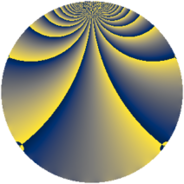# Properties

 Label 1148.2.bkLevel $1148$ Weight $2$ Character orbit 1148.bk Rep. character $\chi_{1148}(37,\cdot)$ Character field $\Q(\zeta_{15})$ Dimension $224$ Sturm bound $336$

# Learn more about

## Defining parameters

 Level: $$N$$ $$=$$ $$1148 = 2^{2} \cdot 7 \cdot 41$$ Weight: $$k$$ $$=$$ $$2$$ Character orbit: $$[\chi]$$ $$=$$ 1148.bk (of order $$15$$ and degree $$8$$) Character conductor: $$\operatorname{cond}(\chi)$$ $$=$$ $$287$$ Character field: $$\Q(\zeta_{15})$$ Sturm bound: $$336$$

## Dimensions

The following table gives the dimensions of various subspaces of $$M_{2}(1148, [\chi])$$.

Total New Old
Modular forms 1392 224 1168
Cusp forms 1296 224 1072
Eisenstein series 96 0 96

## Trace form

 $$224q + 4q^{5} - 116q^{9} + O(q^{10})$$ $$224q + 4q^{5} - 116q^{9} - 4q^{11} - 12q^{15} + 6q^{17} - 4q^{19} + 17q^{21} - 3q^{23} + 32q^{25} + 12q^{27} + 8q^{29} + 4q^{31} + 11q^{33} - 24q^{35} + 11q^{37} + 10q^{39} + 34q^{41} - 28q^{43} - 14q^{45} + 22q^{47} + 56q^{49} - q^{51} - 7q^{53} - 28q^{55} + 44q^{57} - 14q^{59} + 54q^{61} + 18q^{63} + 31q^{65} + 16q^{67} - 96q^{69} + 66q^{71} - 26q^{73} + 39q^{75} - 92q^{77} + 10q^{79} - 80q^{81} + 48q^{83} - 72q^{85} - 22q^{87} + 18q^{89} + 14q^{91} - 40q^{93} + 6q^{95} - 12q^{97} - 48q^{99} + O(q^{100})$$

## Decomposition of $$S_{2}^{\mathrm{new}}(1148, [\chi])$$ into newform subspaces

The newforms in this space have not yet been added to the LMFDB.

## Decomposition of $$S_{2}^{\mathrm{old}}(1148, [\chi])$$ into lower level spaces

$$S_{2}^{\mathrm{old}}(1148, [\chi]) \cong$$ $$S_{2}^{\mathrm{new}}(287, [\chi])$$$$^{\oplus 3}$$$$\oplus$$$$S_{2}^{\mathrm{new}}(574, [\chi])$$$$^{\oplus 2}$$